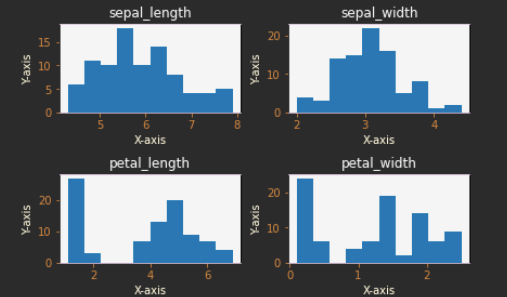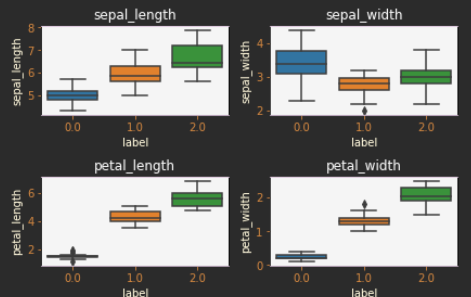Search
•Tim Burns

# Comprehending the Iris Data Set from PySpark

Updated: Jul 4, 2021As I wind my way into Machine Learning, I encountered the "Hello World" of ML Data Sets, the "Iris Data Set."

From the following articles

It is a mistake to try to start machine learning with PySpark. The beginner's view doesn't require distributed processing and fundamental understanding is key to success.

```#%%
print(iris)

#%%
classifier_df = iris.toPandas()
classifier_df.describe()```The values reflect three kinds of Irises in the data set. PySpark requires Double encoding for all text values.

Species Value

Iris Setosa 0.0

Iris Versicolor 1.0

Iris Virginica 2.0

The total count is 150 data points.

Converting to Pandas has the advantage of opening toolsets such as "scikit-learn,"

The coded values convert to 0, 1, 2, 3, representing sepal_length, sepal_width, petal_length, and petal_width.

```#%%
rows = []
column_names = ["sepal_length", "sepal_width", "petal_length", "petal_width"]
columns = None
for row in iris.collect():

feature = row["features"]
if columns is None:
columns = ["label"]
for index in feature.indices:
columns.append(column_names[index])

row = [row["label"]]
row.extend(feature.values)
rows.append(row)

# Build the whole model as a data frame to use in ML
import pandas as pd
iris_data = pd.DataFrame(rows, columns=columns)
iris_data.describe()

```Plotting data is important for intuitive understanding.

```#%%
import matplotlib.pyplot as plt
n_bins = 10
fig, axes = plt.subplots(2, 2)

index = 0
for ax in range(0,2):
for ay in range(0,2):
set_colors(axes[ax,ay])
axes[ax,ay].set_title(columns[index+1], color='whitesmoke')
axes[ax,ay].hist(train[columns[index+1]], bins = n_bins);
axes[ax,ay].set_title(columns[index+1]);
index = index + 1The box plot provides a vivid means of grouping data by density and showing outliers.

Here we use the Seaborn package with plot.

```#%%
import seaborn as sns
fig, axes = plt.subplots(2, 2)
cn = [0.0, 1.0, 2.0]
index = 0
for ax in range(0,2):
for ay in range(0,2):
set_colors(axes[ax,ay])
axes[ax,ay].set_title(columns[index+1], color='whitesmoke')
sns.boxplot(x = 'label', y = columns[index+1], data = train, order = cn, ax = axes[ax,ay]);
index = index + 1

# add some spacing between subplots